# Areas related to Cirles-Sample Papers

Class X Math
Test for Areas Related to Circles
Total Marks : 25
Total Time : 30 mins
1.   PQRS is a diameter of a circle of radius 6 cm. The equal lengths PQ, QR and RS are drawn on PQ and QS as diameters, as shown in figure. FInd the perimeter of the shaded region.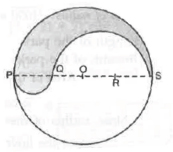(2 marks)
2.   A sheet of paper is in the form of a rectangle ABCD in which AB = 40 cm, and BC = 28 cm. A semicirclular portion with BC as diameter is cut off. Find the area of the remaining paper.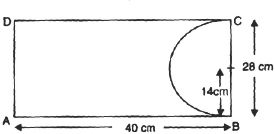(2 marks)
3.   Find the area of the shaded region in the figure, if ABCD is a square of side 14 cm and APD and BPC are semi-circles.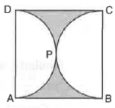(2 marks)
4.   Find the area of the shaded region of the following figure, if the diameter of the circle with centre O is 28 cm and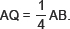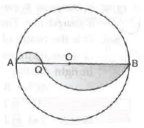(3 marks)
5.   PQRS is a square land of side 28 m. Two semi-circular grass covered postions are to be made on two of its opposite sides as shown in the figure. How much area will be left uncovered?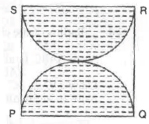(3 marks)
6.   In the given figure, O is the centre of a circular arc and AOB is a straight line. Find the perimeter of the shaded region.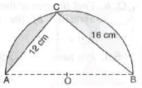(2 marks)
7.   In a circular table cover of radius 70 cm, a design is formed leaving an equilateral triangle ABC in the middle as shown in teh figure. Find the total area of the design.
(4 marks)
8.   Calculate the area other than the area common between two quadrants of the circles of radius 16 cm each,. which is shown as the shaded region in the figure.
(4 marks)
9.   In teh figure PQ = 24 cm, PR = 7 cm and O is the centre of the circle. Find the area of shaded region.
(3 marks)